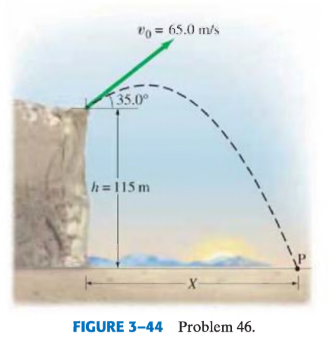# Problem: A projectile is shot from the edge of a cliff 115 m above ground level with an initial speed of 65.0 m/s at an angle of 35.0° with the horizontal, as shown in Fig. 3-44.(a) Determine the time taken by the projectile to hit point P at ground level.(b) Determine the distance X of point P from the base of the vertical cliff.At the instant just before the projectile hits point P, find:(c) the horizontal and the vertical components of its velocity,(d) the magnitude of the velocity,(e) the angle made by the velocity vector with the horizontal.(f) Find the maximum height above the cliff top reached by the projectile.

###### FREE Expert Solution
88% (262 ratings)
###### Problem Details

A projectile is shot from the edge of a cliff 115 m above ground level with an initial speed of 65.0 m/s at an angle of 35.0° with the horizontal, as shown in Fig. 3-44.
(a) Determine the time taken by the projectile to hit point P at ground level.
(b) Determine the distance X of point P from the base of the vertical cliff.
At the instant just before the projectile hits point P, find:
(c) the horizontal and the vertical components of its velocity,
(d) the magnitude of the velocity,
(e) the angle made by the velocity vector with the horizontal.
(f) Find the maximum height above the cliff top reached by the projectile.